Home > GC > Chapter 8 > Lesson 8.3.3 > Problem8-115

8-115.
1. Christie has tied a string that is 24 cm long into a closed loop, like the one below. Homework Help ✎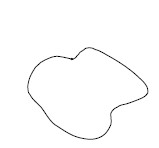1. She decided to form an equilateral triangle with her string. What is the area of the triangle?

2. She then forms a square with the same loop of string. What is the area of the square? Is it more or less than the equilateral triangle she created in part (a)?

3. If she forms a regular hexagon with her string, what would be its area? Compare this area with the areas of the square and equilateral triangle from parts (a) and (b).

4. What shape should Christie form to enclose the greatest area?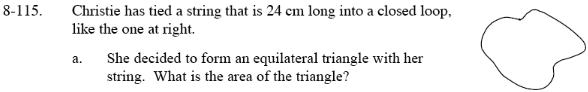$\text{The length of each side would be }=\frac{24}{3}.$

Use the Pythagorean Theorem to solve for the height of the triangle, which will then allow you to find its area.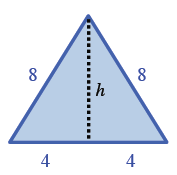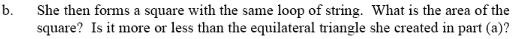$\text{The length of each side would be }=\frac{24}{4}.\ \ \text{Find the square's area by multiplying its length by its width}.$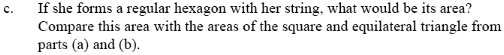$\text{The length of each side would be }=\frac{24}{6}.\ \ \text{Find the area of the hexagon by dividing it into triangles}.$

Note that when one of the triangle is split in two, it is a special 30°-60°-90° right triangle. Use this information to solve for the height of the triangle, and the find the area of the triangle and the hexagon.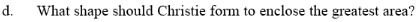The more sides a shape has, the larger its area is.

A circle would have the greatest area, because it is like a polygon with infinite sides.